## Simplifying Fractions Worksheet and Template

I’ve dubbed this school year “The Year of Fractions” for my daughter.  She’s currently in Singapore Math 4A and she’s been working with fractions almost since the beginning of school.  She’s been adding and subtracting them, writing them as mixed numbers, changing improper fractions to mixed numbers, and simplifying them to boot.

When it came to simplifying fractions, I was a bit worried.  You really have to analyze the numbers in the numerator and denominator to decide if the fraction can be reduced any further and sometimes that’s a hard concept for kids. I thought it would be helpful to have a guide for her to use at the beginning.  I created this flip chart that we put in her math notebook that walked her through six questions to consider when she’s asked to simplify a fraction.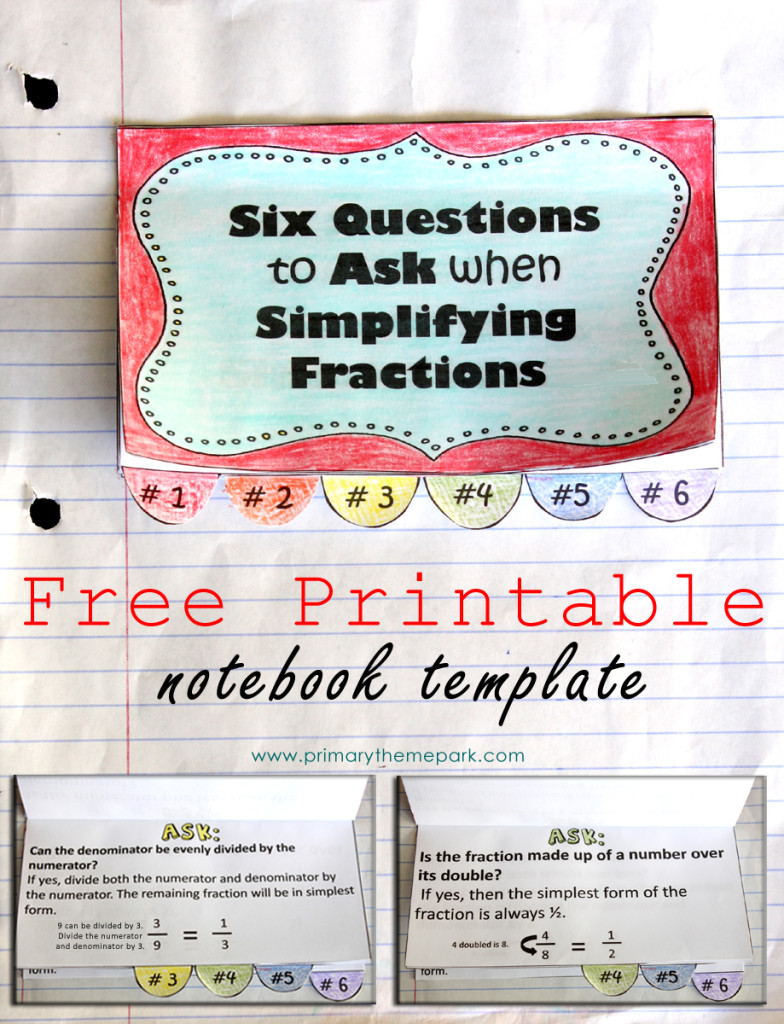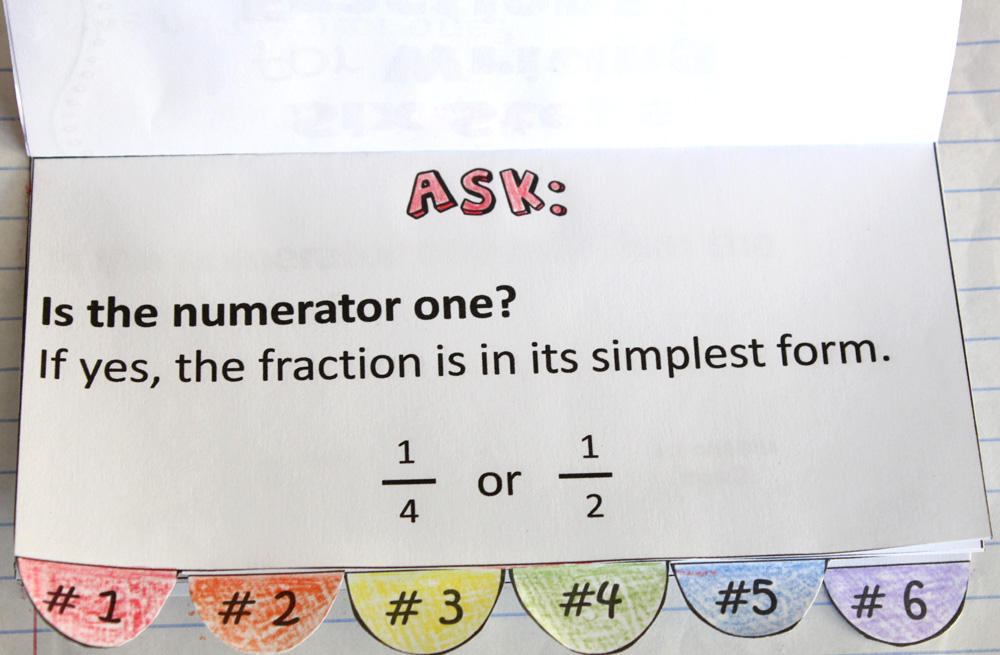1.  If the numerator of the fraction is one, it cannot be simplified any further.  This is because the only factor of one is one, so if you divide the numerator and denominator by one, you will have the exact same fraction you started with.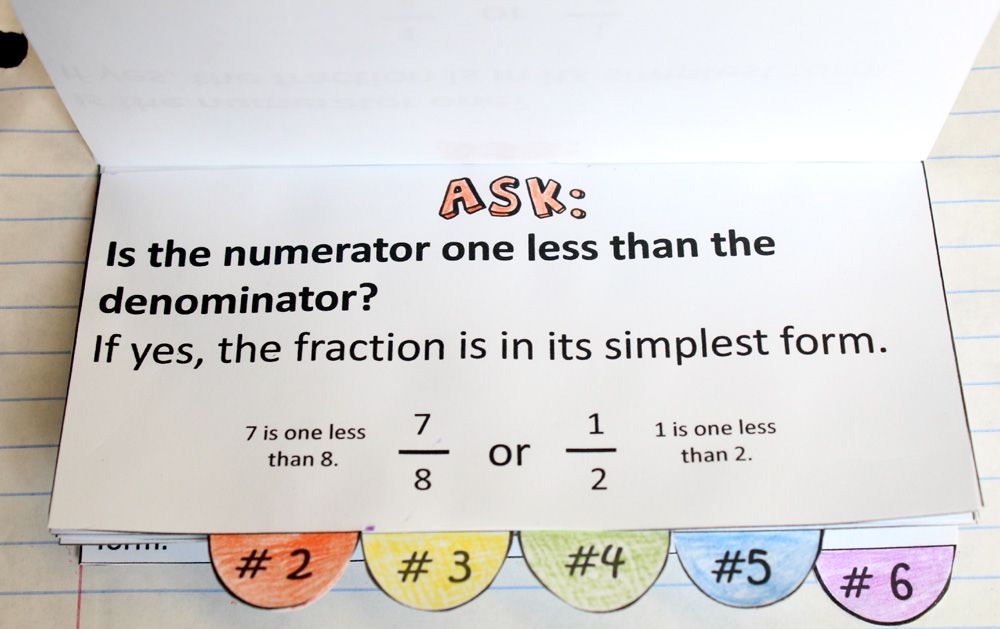2. If the numerator is one less than the denominator, the only factor they will have in common is one.  So, again, if you divide the numerator and denominator both by one, you will have the original fraction.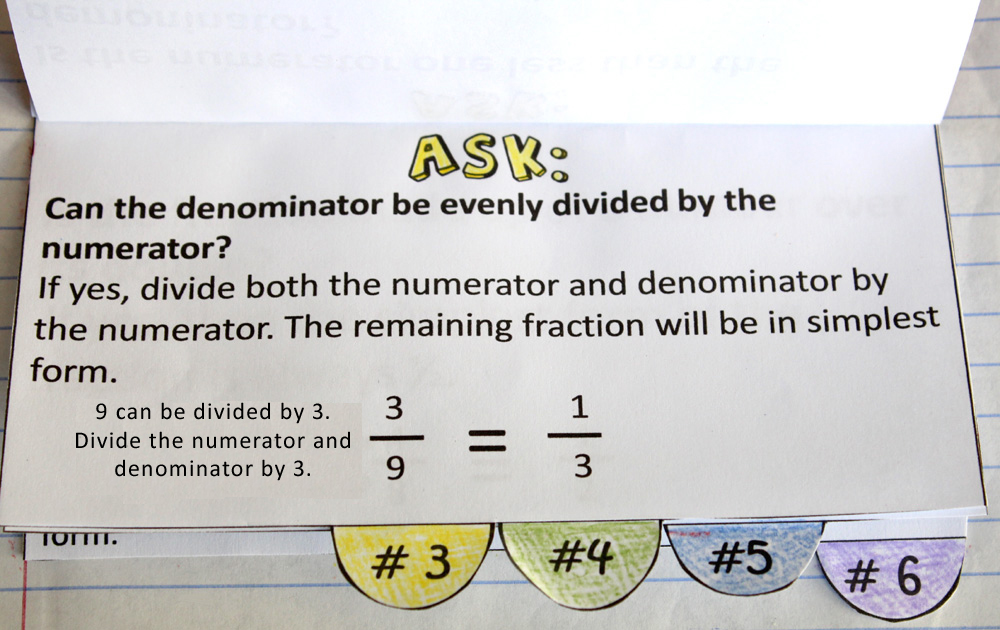3.  If the denominator can be evenly divided by the numerator, the number in the numerator is a common factor of both.  Dividing by the numerator will result in a fraction with a numerator of one, and the reduced fraction will be in simplest form.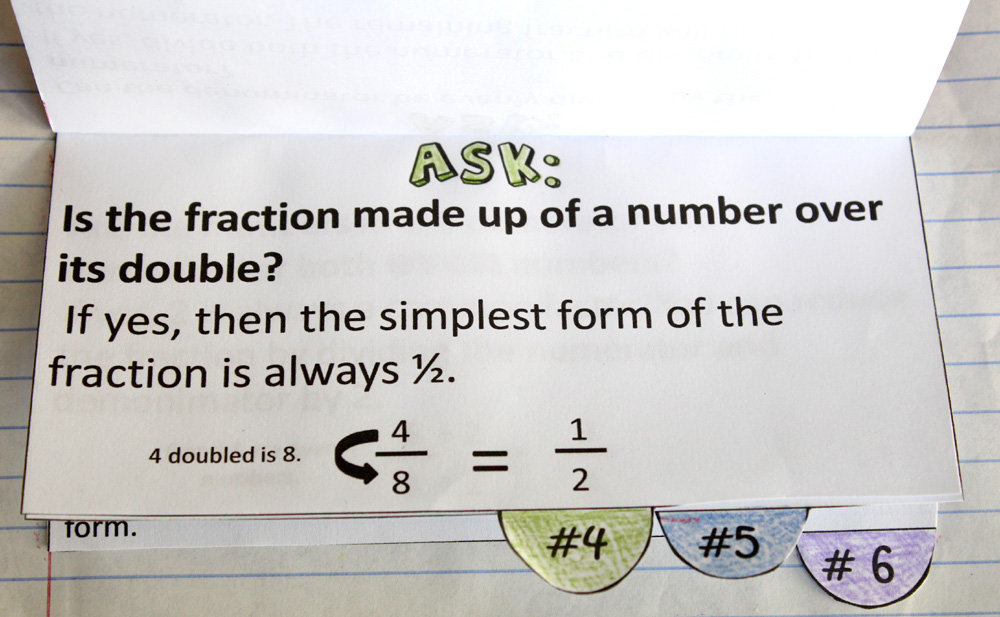4.  If the numerator is half the amount of the denominator, then the fraction is essentially one half.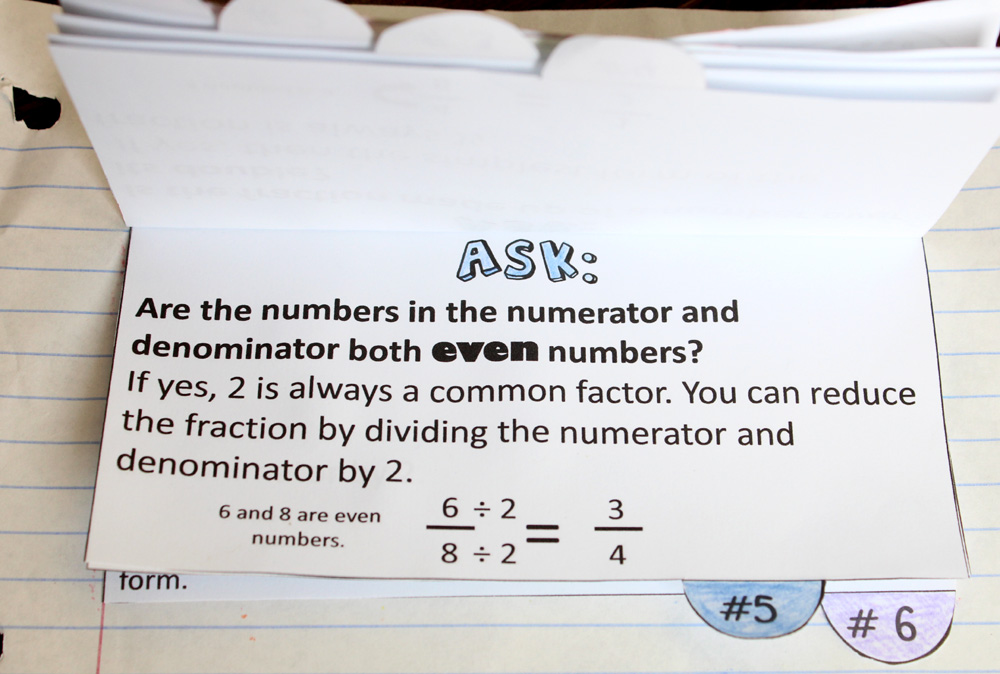5.  All even numbers are divisible by two.  If after reducing the fractions there are still even numbers in both the numerator and denominator, keep dividing both by two until you cannot evenly divide by two anymore.  For example, in the fraction 12/20, if you divide both numbers by two, the remaining fraction is 6/10.  6/10 is not in simplest form because 6 and 10 are still both even numbers.  Divide both 6 and 10 by two again to find the simplest form is 3/5.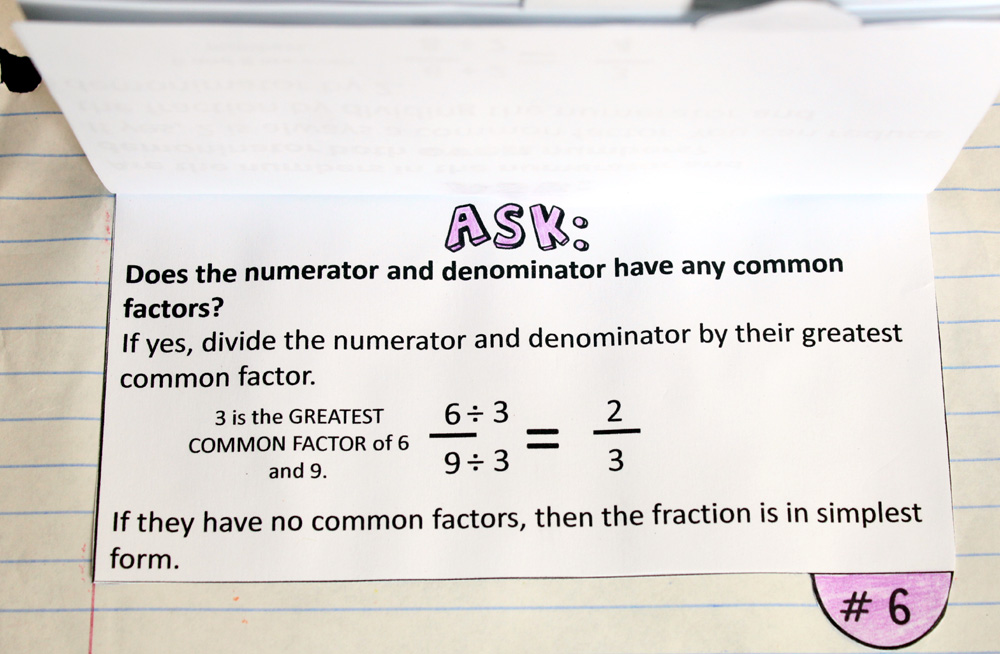6. Dividing the numerator and denominator by their greatest common factor will reduce the fraction to its simplest form. If both the numerator and denominator have no common factors other than one, then the fraction is already in simplest form.

When we first began simplifying fractions, we would flip through this chart and ask each question of every fraction.  These questions helped walk her through analyzing the fraction to see what steps she needed to take in order to simplify it.  After a couple of days of practice, she could look at a fraction, immediately identify which question it answered, and simplify it.  I was amazed at how just breaking down the thinking process into questions turned simplifying fractions into a breeze!

Since this little flip chart made our lives a little bit easier, I wanted to share it with you!  Glue it onto notebook paper or place it in an interactive math notebook.  I also compiled these 6 questions onto just one page to create this simplifying fractions worksheet in case that’s more helpful for you than a flip chart.  I hope these resources help your students sail through simplifying fractions, too!## The Snowy Day Activities

1.kaz says:

Where can I find a template for theinteractive notebook materials. I think its great stuff.

2.Stephanie says:

Hi, there! Right above the last picture there is a sentence that says, “You can download it by clicking here”. Click on the word “here” in that sentence and it should download the file for you. Once the file opens, you can save it to your computer and then print it out. I hope that helps! 🙂

3.Bonnie says:

Hi, I saw your post on Pinterest and I really love this strategy for teaching simplifying fractions. Great job! I am using this with my fourth graders and they really like the way the questions help them to work out the fractions.

1.Stephanie says:

Thanks, Bonnie! I’m so happy to hear that these questions are helping your students with fractions!

4.frances says:

Thank you – I love it!

5.Liz says:

Thank you! This looks just perfect to use with my 4th and 5th grade class.

6.Maria Brown says:

Thank you! Great strategy, will use it with my fifth graders!

7.jo says:

Omg, I am tutoring my mom on fractions right now. I made a document on my stupid phone on Office Suite-PLEASE tell everyone not to ever use or even look at-it was a very detailed, step-by-step guide on interchanging improper fractions and mixed numbers. It cut half my document off and had the title in red in the middle of the page. I’ve been on here browsing for instructional, step-by-step worksheets on fractions and this is the first one I found. Thank you so much!